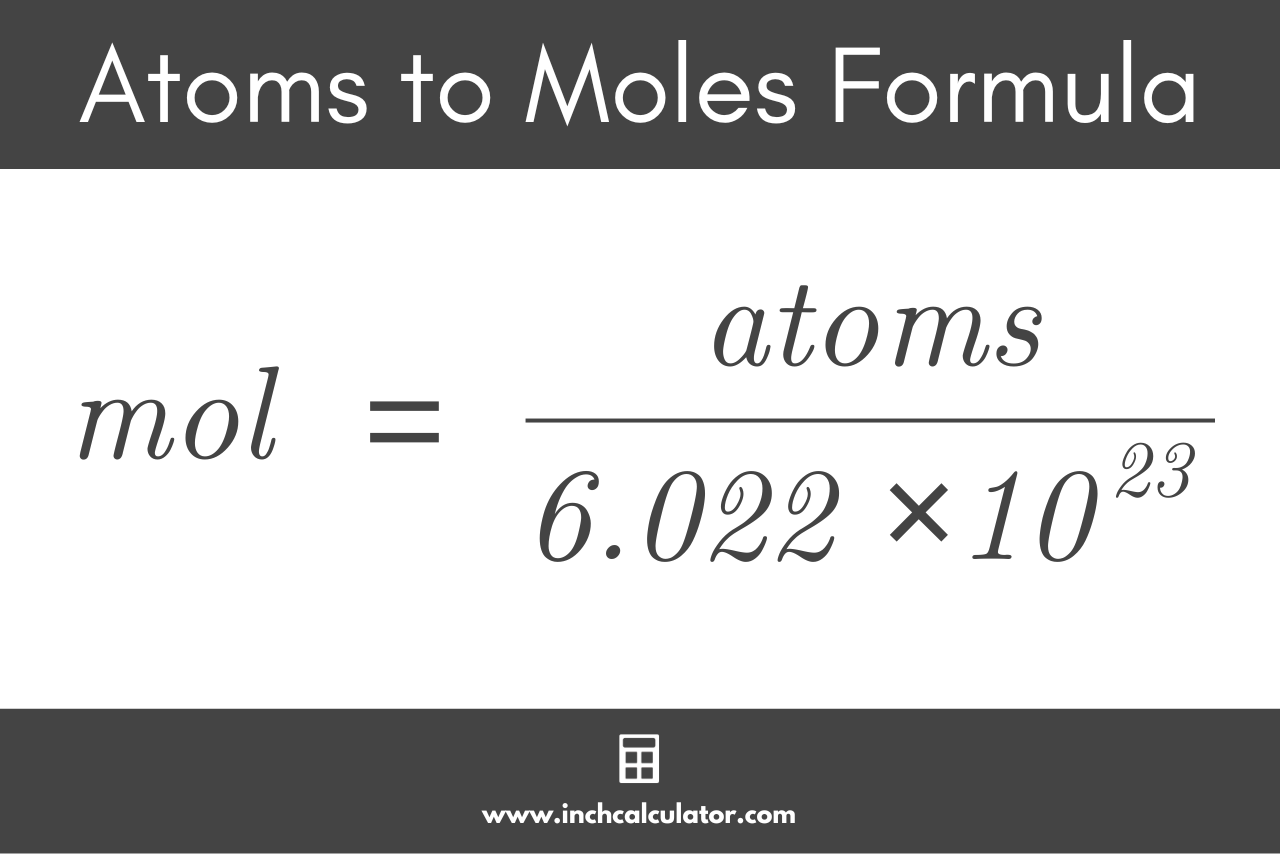# Atoms to Moles Calculator

Convert atoms to moles or vice-versa using the conversion calculator below.

## Results:

moles
Learn how we calculated this below

## How to Convert Atoms to Moles

In chemistry, the SI base unit for the quantity (number of atoms, ions, or molecules) of a pure substance is the mole, which is abbreviated mol. To convert the number of atoms of a pure substance to moles, you’ll use a simple formula based on the number of molecules in a mole, which is a constant.

Moles are typically used instead of weight or volume in chemistry, because writing chemical equations in terms of moles shows the relationships between the amounts of the reactants and the products, and it is a quantity large enough to easily measure. For example: 2NaCl = 2Na + Cl2.

Avogadro’s constant (often called Avogadro’s number) defines the number of particles of a substance, such as atoms, molecules, or ions, that make up a mole. Avogadro’s number is 6.02214076 × 1023.

Avogadro’s constant is so large that it’s almost always referred to using scientific notation. It is named for Amedeo Avogadro, who defined this value in the early 19th century and stated that the volume of any gas is proportional to the number of atoms and molecules within.

### Atoms to Moles Formula

Using Avogadro’s constant, the formula to convert atoms to moles is:

mol = atoms ÷ 6.02214076 × 1023

Thus, the amount of a purse substance in moles is equal to the number of atoms divided by Avogadro’s constant, or 6.02214076 × 1023.## How to Convert Moles to Atoms

To convert moles to atoms, the formula above can be reversed.

### Moles to Atoms Formula

atoms = mol × 6.02214076 × 1023

Thus, the number of atoms in a substance is equal to the quantity in moles times Avogadro’s constant.

Did you know that you can convert liters to moles or grams to moles using a similar formula? These conversions are widely used in the lab to prepare the correct quantities for a chemical reaction.

## References

1. International Bureau of Weights and Measures, SI base unit: mole (mol), https://www.bipm.org/en/si-base-units/mole